# How to Calculate the Midrange in Excel?

• Last Updated : 30 Jul, 2021

MS Excel is a spreadsheet developed by the company Microsoft. Excel provides various kinds of functions and we can insert the data in form of rows and columns and perform operations on the data and yield the results we desired.

The Midrange of the dataset in other terms can be specified as average or mean. the Midrange is also known as the measure of center in statistics.

Attention reader! Don’t stop learning now. If you are an Excel beginner (or an intermediate) and want to learn Excel, Geeksforgeeks brings the perfect course for you to start, Diving Into Excel

`Midrange of any dataset can be calculated as follows: (largest value + smallest value) / 2`

In order to calculate using formulas of excel we follow 3 steps:

• Calculate Min value
• Calculate Max value
• Apply midrange formula

Consider the following data i.e. marks obtained by students of a class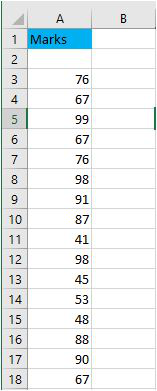Sample data

To find the midrange of the given data we will follow the 3 steps

Step 1:Calculate the min value of the data by using the min function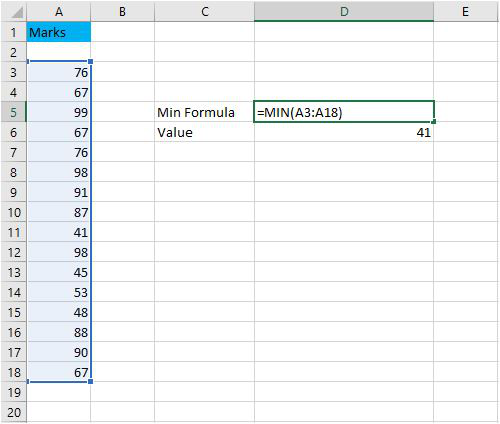Min Value

Step 2:Calculate the max value of the data by using the max function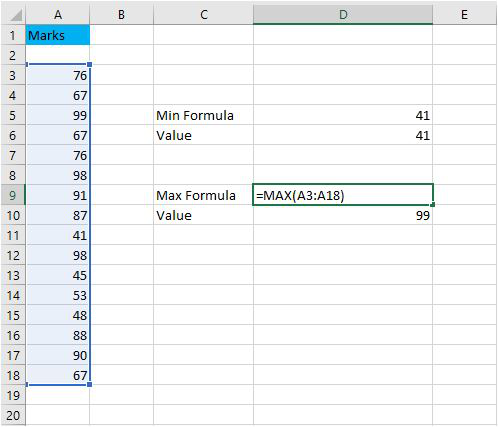Max Value

Step 3:Now apply the midrange formula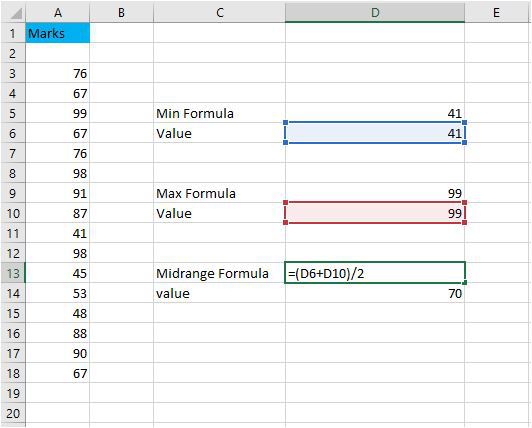Midrange of the data

Finally, we get the midrange of the data as 70.

### Drawbacks:

• The midrange deviates hugely when there are outliers in our data
• We need to find first the maximum and minimum value to the data in order to find the midrange
• The midrange sometimes differs from mean and median hugely.

For example in our data, consider another field with the value of 1000 then the midrange will be 520.5 which is far away from the original midrange of 70. So this shows how large the midrange deviates from the outliers.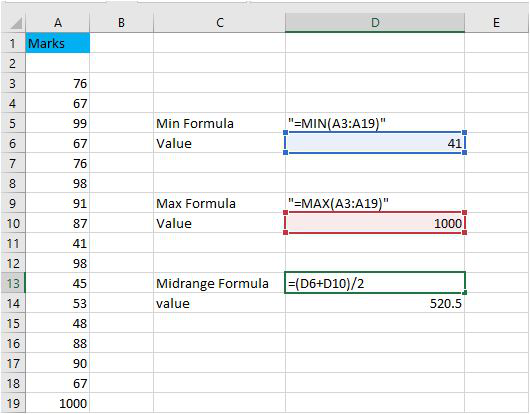### Alternatives:

Instead of finding the midrange, we can directly calculate the mean, median of the data  which points to the average or the midpoint of the data

Mean: It is the average value of the data set. It is calculated by the formula

`Mean = Sum of all the observations/Total number of Observations`

In excel we can directly compute the mean by using the average function.

Median: It is the middle value of the dataset. We need to first arrange the dataset in either non-increasing or non-decreasing order and select the middlemost element. In case there are two middlemost elements we compute the average of two.

In excel we can compute it by using the median function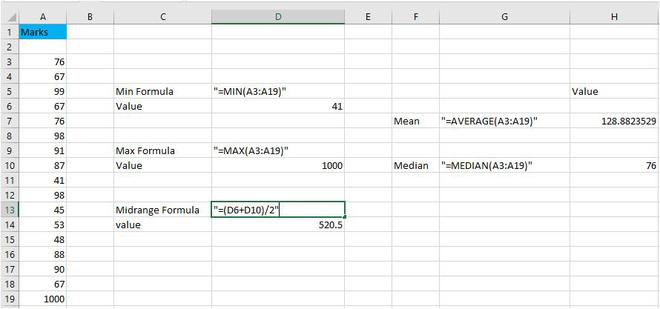This image gives the mean, median and midrange of the dataset.

My Personal Notes arrow_drop_up